## UNDERSTANDING THE PROBLEM

After solving a few machine learning problems now, one thing which I have realized is that data cleaning and feature engineering is the most important part in ANY problem. Checking the correlation and the impact, looking to increase a few dependent variables which might be having a high impact on the response are critical for a good model. It is easy to apply various models, but to fine tune the model and mold the data accordingly is the difficult part.

Problem Statement:

In the problem of Bike Sharing Demand, we are given the total number of bike rentals for each hour for the 1st to 19th of every month for two years and we need to predict the number of rentals for the next 11 days for each month.
Luckily the problem statement is simple here and data is pretty nicely explained.

The data:

For every hour we are given the season, temperature, humidity, weather condition and wind speed. Also, another important parameter here is the day type i.e. holiday, working day or a weekend. Though we need to predict the total rentals for an hour, we also have the data for how many of the total rentals are from registered users and how many are from casual users.

Initial Observation

• All the factors in test are in train. (Good news)
• Would need to check if predicting registered rentals and casual rentals separately and then adding them would make more sense or not
• Registered users are the ones using more on working days and casual users are the ones using on holidays
• We need to predict a continuous variable using factors and numeric values, so checking if there is are outliers in the response variable would be a good option. (Box plot can be used (check Visualization tab), and if there are outliers then we can take log of the rentals)
• Correlations could play a big role here (atemp and temp, the correlation is 0.98)
make much sense.

Feature Engineering

• Firstly, the most obvious thing to do here would be to split the date and time. Make new variables for month, year, date and time.
• With the date, we can even figure out the day of the week and combine weekends, working days and holidays using the previously available data.
• We could group the time into peak hours for registered and casual users. I have used rpart to do this for me.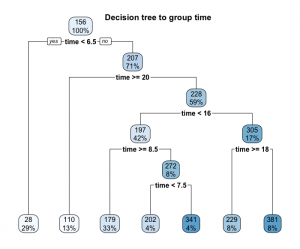• Similar trees could be created to group temperature as well
• Wind speed is 0 is many cases, which we can consider as missing. So we can use random forest to predict these values
• If we include month, a problem can be that the rentals in for example January 2011 and January 2012 may not be related. But the sales of the previous months would incorporate the growth, hence including quarters in our model would make sense

Now let us visualize the data as much as possible and then try to develop a model to predict.

## EXPLORATORY DATA ANALYTICS

Now, let’s visualize the data of bike sharing demand we have and see if it really makes any sense and if we can get some more insight.

• As previously mentioned, let us draw the boxplot for count to check for outliers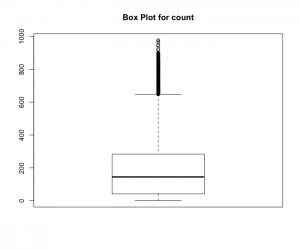• Since there are a high number of outliers, let us take log of count and recheck if that helps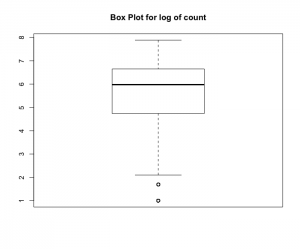• Another assumption we had made was that registered users use more on working days to go for work and casual users would use more on holidays.
The following graphs perfectly explain this trend.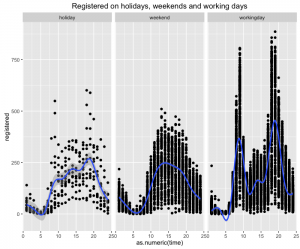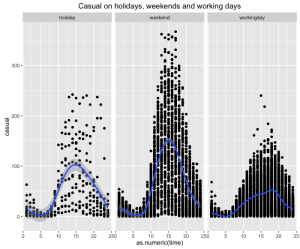• Using random forests we can see the relative importance of various parameters in the initial given data and then build new feature accordingly.
The next two graphs show how the partial plot for the variables vary and their importance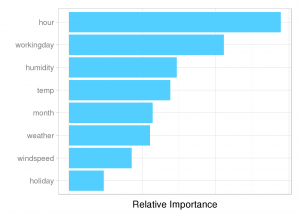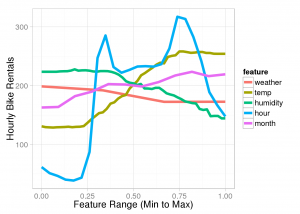## PREDICTING BIKE SHARING DEMAND

Here we have factors as well as numeric variables in this prediction and we have to predict continuous variable. Had tried regression on this, which is the first method which comes in mind, but that did not work well. So here is the code using random forest in R which got me in the top 10% percent OF Bike Sharing Demand problem in Kaggle.

Those who do not remember the past are condemned to repeat it.George Santayana